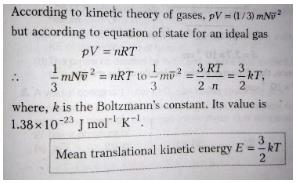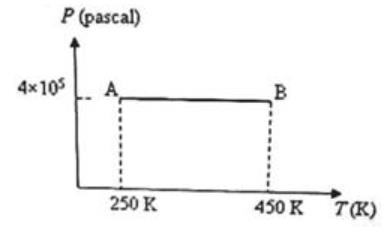Courses

# Test: Behaviour of Ideal Gases

## 10 Questions MCQ Test Physics Class 11 | Test: Behaviour of Ideal Gases

Description
This mock test of Test: Behaviour of Ideal Gases for JEE helps you for every JEE entrance exam. This contains 10 Multiple Choice Questions for JEE Test: Behaviour of Ideal Gases (mcq) to study with solutions a complete question bank. The solved questions answers in this Test: Behaviour of Ideal Gases quiz give you a good mix of easy questions and tough questions. JEE students definitely take this Test: Behaviour of Ideal Gases exercise for a better result in the exam. You can find other Test: Behaviour of Ideal Gases extra questions, long questions & short questions for JEE on EduRev as well by searching above.
QUESTION: 1

### In the case of real gases, the equation of state, PV = RT (where P, V and T are respectively the pressure, volume and absolute temperature), is strictly satisfied only if corrections are applied to the measured pressure P and the measured volume V. The corrections for P and V arise respectively due to

Solution:

According to kinetic theory of gases there is no intermolecular force of attraction between the molecule of gases but its not true as gases liquifise, at high pressure molecular interaction starts due to which pressure on the walls decreases as molecule dragged back so pressure exerted by real gas is less than ideal gas, due to repulsive forces molecule behave as small spheres and hence volume decreases

QUESTION: 2

### Which one of the following quantities can be zero on an average for the molecules of an ideal gas in equilibrium?

Solution:

In case of ideal gases the average velocity is always zero. Hence the average momentum is zero.
Whereas average speed is non- zero so the kinetic energy is also non-zero,  as these two are scalar quantities.

QUESTION: 3

### Two specific heats of a perfect gas are related by:

Solution:

When cp & cv are given calorie & R in Joule then Cp – Cv = (R/J)

QUESTION: 4

One mole of any substance at any temperature, pressure or volume always contains ________ molecules.

Solution:

1 mole = 6.02 x 1023

QUESTION: 5

Oxygen and nitrogen in two enclosures have the same mass, volume and pressure. The ratio of the temperature of oxygen to that of nitrogen is:

Solution:

For same mass the ratio of moles of oxygen to that of nitrogen is 14:16 = 7:8
And we know that PV = nRT
Hence as V and P are also same, ratio of temperature of oxygen to that of nitrogen is inverse of the ratio of moles that is 8:7

QUESTION: 6

When the temperature goes up, the pressure inside a rigid container will _____.

Solution:

The pressure law states that for a constant volume of gas in a sealed container the temperature of the gas is directly proportional to its pressure. This can be easily understood by visualising the particles of gas in the container moving with a greater energy when the temperature is increased.

QUESTION: 7

Four moles of an ideal diatomic gas is heated at constant volume from 20° C to 30° C. The molar specific heat of the gas at constant pressure (Cp) is 30.3 Jmol-1K-1 and the universal gas constant (R) is 8.3 Jmol-1K-1. The increase in internal energy of the gas is

Solution:

The value of Cp is 30.3
and as Cp-Cv = R(8.3)
hence Cv = 30.3-8.3
Cv is 22
change in internal energy = no of moles × Cv × change in temperature
hence
change in internal energy = 22 × 4 × 10
= 880j
Hence Option D is correct.

QUESTION: 8

A region of the earth’s atmosphere contains n molecules (treated as ideal gas molecules) per unit volume. The temperature of air in the region is T. If k represents Boltzmann’s constant and R represents universal gas constant, the pressure of air in the region is

Solution:

PV = nRT
Where n = number of moles = m/NA
So, P = (m/V)(R/NA)T
Also, we know that R/NA = k
So, P = nkT

QUESTION: 9

The average kinetic energy of translation of a molecule of an ideal gas at temperature T is:

Solution:QUESTION: 10

Three moles of an ideal monoatomic gas is initially in the state A shown in the adjoining pressure-temperature graph. It is taken to state B without changing its pressure. If R is the universal gas constant, the work done by the gas in this process isSolution:

The work done by the gas in taking it from state A to state B = PΔV where ΔW is the increase in volume at constant pressure P.

We have PV = μRT where p is the number of moles in the sample of the gas and R is the universal gas constant.

Therefore we have PΔV = μR ΔT = 3 xR(450 - 250) = 600R